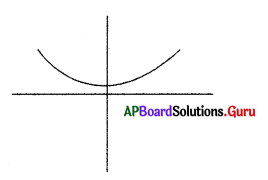Practice the AP 10th Class Maths Bits with Answers Chapter 5 Quadratic Equations on a regular basis so that you can attempt exams with utmost confidence.

## AP State Syllabus 10th Class Maths Bits 5th Lesson Quadratic Equations with Answers

Question 1.
If sin α, cos α are roots of the equation ax2 + bx + c = 0, then ………………
A) a2 + b2 – 2ac = 0
B) (a + c)2 = b2 + c2
C) a2 – b2 + 2ac = 0
D) (a + c)2 = b2 – c2
D) (a + c)2 = b2 – c2

Question 2.
If px2 + qx + r = 0 has equal roots , then r = ……………….
A) $$\frac{\mathrm{q}^{2}}{2 \mathrm{p}}$$
B) $$\frac{\mathrm{q}}{2 \mathrm{p}}$$
C) $$\frac{-q^{2}}{4 p}$$
D) $$\frac{q^{2}}{4 p}$$
D) $$\frac{q^{2}}{4 p}$$Question 3.
If the equations x2 + bx + c = 0, x2 + cx + b = 0 [b ≠ c] have a common root, then …………………
A) b + c = 0
B) b + c = 1
C) b + c + 1 = 0
D) None of these
C) b + c + 1 = 0

Question 4.
The roots of x – $$\frac{3}{x}$$ = 2 are
A) 3, 2
B) 3,-2
C) 3, 1
D) 3, – 1
D) 3, – 1

Question 5.
The roots of the quadratic equation 2x2 -2√2x + 1 = 0 are ……………….
A) √2, $$\frac{1}{\sqrt{2}}$$
B) $$\frac{1}{2}, \frac{1}{2}$$
C) $$\frac{1}{\sqrt{2}}, \frac{1}{\sqrt{2}}$$
D) √2, √2
C) $$\frac{1}{\sqrt{2}}, \frac{1}{\sqrt{2}}$$

Question 6.
If α, β are the roots of x2 + 2x + 5 = 0 then $$\frac{\alpha}{\beta}+\frac{\beta}{\alpha}$$ =
A) $$\frac{6}{5}$$
B) $$\frac{4}{5}$$
C) $$-\frac{6}{5}$$
D) $$-\frac{4}{5}$$
C) $$-\frac{6}{5}$$

Question 7.
If the roots of a(b – c)x2 + b(c – a) x + c(a – b) = 0 are equal, then a, b, c are in
A) AP
B) GP
C) HP
D) None
C) HP

Question 8.
The roots of are $$\frac{1}{x+4}-\frac{1}{x-7}=\frac{11}{30}$$
A) -1, 2
B) 1, 2
C) 1, -2
D) -1, -2
B) 1, 2

Question 9.
If A is the solution set of x2 – 5x + 6 = 0 and B is the solution set of x – $$\sqrt{3 x-6}=$$ = 2, then A ∩ B =
A) Φ
B) A
C) B
D) {2}
D) {2}

Question 10.
If the sum of the squares of the roots of x2 + px – 3 = 0 is 10, then p =
A) ± 2
B) ± 3
C) ± 5
D) ± 6
A) ± 2Question 11.
If one root of x2 – 8x + 13 = 0 is 4 + √3,then the other root is …………….
A) 2 + √3
B) 2 – √3
C) -4 + √3
D) 4 – √3
D) 4 – √3

Question 12.
If α and β are the roots of a quadratic a P equation x2 – px + q = 0, then $$\frac{\alpha}{\beta}+\frac{\beta}{\alpha}$$ =
A) $$\frac{p^{2}-2 q}{q}$$
B) $$\frac{p^{2}+2 q}{q}$$
C) $$\frac{p^{2}-q}{q}$$
D) $$\frac{p^{2}+q}{q}$$
D) $$\frac{p^{2}+q}{q}$$

Question 13.
If x = $$\sqrt{3+\sqrt{3+\sqrt{3+\ldots \ldots \infty} \infty}}$$ then
A) x2 – x + 3 = 0
B) x2 + x + 3 = 0
C) x2 – x – 3 = 0
D) x2 + x – 3 = 0
C) x2– x – 3 = 0

Question 14.
If the difference of two numbers is 5 and their product is 84, then the numbers
A) 14, 6
B) 12, 7
C) 21, 4
D) 14, 9
B) 12, 7

Question 15.
$$\sqrt{6+\sqrt{6+\sqrt{6+\ldots \ldots \infty} \infty}}$$ =
A) 1
B) 2
C) 3
D) 4
C) 3

Question 16.
If x + $$\frac{1}{x}$$ = 2, then x2 + $$\frac{1}{x^{2}}$$ =
A) 0
B) 2
C) 4
D) 8
B) 2

Question 17.
The discriminant of the quadratic equation ax2 + bx + c = 0is
A) b2 – 4ac
B) a2 – 4bc
C) c2 – 4ab
D) None
A) b2 – 4acQuestion 18.
A ball is thrown vertically upward from the ground. The distance s in t seconds is given by s = 4t2 + t – 3. After how many seconds does the ball come to rest?
A) 2s
B) 1s
C) $$\frac{3}{4}$$s
D) $$\frac{4}{3}$$s
A) 2s

Question 19.
The product of the roots of √2x2 – 10x + 5√2 = 0 is
A) 5√2
B) 5
C) 2√5
D) 2
A) 5√2

Question 20.
The discriminant of $$\sqrt{x^{2}-5 x-1}$$ = 2 is
A) 21
B) 23
C) 27
D) 45
C) 27

Question 21.
The equation x2 + x + 1 = 0 has
A) real equal roots
B) no real roots
C) real and unequal roots
D) All of the above a
B) no real roots

Question 22.
If $$\frac{\mathbf{x}}{\mathbf{a}-\mathbf{b}}=\frac{\mathbf{a}}{\mathbf{x}-\mathbf{b}}$$ then x = …………….
A) (b – a), -a
B) (b – a), $$\frac{1}{a}$$
C) (a – b), b2
D) (b – a), a
D) (b – a), a

Question 23.
The discriminant of the equation x2 + 4x + 2 = 0 is ……………….
A) 8
B) -8
C) 10
D) 4
A) 8

Question 24.
The discriminant of the equation px2 + qx + r = 0 is …………………..
A) q2 + 4pr
B) q2 – 4pr
C) p2 – 4qr
D) r2 – 4pq
B) q2 – 4prQuestion 25.
The degree of a quadratic equation is
A) 1
B) 0
C) 2
D) 3
C) 2

Question 26.
In a quadratic equation ax2 + bx + c = 0, if b2 – 4ac > 0, then the roots are ……………….
A) real arid distinct
B) real and equal
C) imaginary
D) none
A) real arid distinct

Question 27.
A quadratic equation, whose roots are 2 + √3 and 2 – √3 = ……………….
A) x2 – x – 4 = 0
B) x2 – 4x + 1 = 0
C) x2 + 4x + 3 = 0
D) x2 + x – 3 = 0
B) x2 – 4x + 1 = 0

Question 28.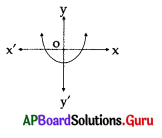A) b2 – 4ac >0
B) b2 – 4ac = 0
C) b2 – 4ac < 0
D) None of the given
A) b2 – 4ac >0

Question 29.
If $$\sqrt{x}+\frac{58}{\sqrt{x}}$$ = 31, then x =
A) 529
B) 933
C) 729
D) 841
D) 841

Question 30.
Which one of the following figures shows the quadratic equation
ax2 + bx + c = 0 (a ≠ 0) having distinct roots ?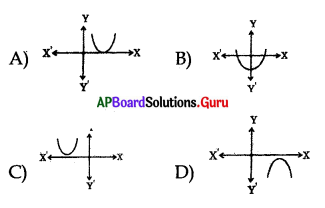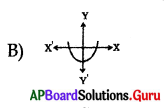Question 31.
If 1 is a common root of ax2 + ax + 2 = 0 and x2 + x + b = 0, then a. b = ………………
A) 2
B) -2
C) 3
D) -3
A) 2Question 32.
Find the zeros of the quadratic polynomial x2 – 5x – 14.
A) (+7,-2)
B) (-7,-2)
C) (-7, +2)
D) (7, 2)
A) (+7,-2)

Question 33.
If one root of the quadratic equation x2 – 4x + 1 = 0 is 2 + √3, then the other
root is ……………………
A) 7 + √3
B) 7 – 4√3
C) 1 + √3
D) 2 – √3
D) 2 – √3

Question 34.
If α, β are the roots of x2 – 10x + 9 = 0, then | α – β|=
A) 9
B) 8
C) -10
D) 10
B) 8

Question 35.
The number of diagonals in a polygon having ‘n’ sides is ………………..
A) $$\frac{n(n+1)}{2}$$
B) $$\frac{n(n-1)}{2}$$
C) $$\frac{n(n-3)}{2}$$
D) $$\frac{n(n+3)}{2}$$
C) $$\frac{n(n-3)}{2}$$

Question 36.
If x2– px + q = 0(p,q ∈ Rand p ≠ 0,q ≠ 0) has distinct real roots, then………………
A) p2 < 4q
B) p2 > 4q
C) p2 = 4q
D) p2 + 4q = 0
B) p2 > 4q

Question 37.
If 5 is a root of x2 – (K – 1)x + 10 = 0, then the value of K is
A) -8
B) 7
C) 8
D) 12
C) 8Question 38.
Sum of the roots of x2 – 16 = 0 is
A) $$\frac{1}{16}$$
B) 1
C) 0
D) 16
C) 0

Question 39.
The sum of squares of the roots of x2 + 8x + 15 = 0 is …………………
A) 30
B) 34
C) 40
D) 44
B) 34

Question 40.
The value of $$\sqrt{2+\sqrt{2+\sqrt{2+\ldots \ldots \ldots}}}$$ is……………….
A) 3
B) 4
C) 2
D) 8
C) 2

Question 41.
The roots of (b – c) x2 + (c – a) x + (a – b) = 0 are equal and kb = a + c, then the value of k is………………..
A) 1
B) 2
C) 3
D) 4
B) 2

Question 42.
The roots of x – $$\frac{3}{x}$$ = 2 are
A) 1, 3
B) 3, -1
C) 2, 2
D) 1, 2
B) 3, -1

Question 43.
If one root of 3x2 + 2x + k = 0 is the reciprocal of the other, then the value of
k is
A) 3
B) -3
C) 2
D) 6
A) 3

Question 44.
If the roots of 2x2 + kx + 3 = 0 are real and equal, then the value of k is
A) ± 2√6
B) ± 6√2
C) ± 4
D) ± 5
C) ± 4Question 45.
The quadratic equation px2 + qx + r = 0 has imaginary roots, if ………………
A) q2 > 4 pr
B) q2 < 4 pr
C) q2 = 4 pr
D) p = q + r
B) q2 < 4 pr

Question 46.
Which of the following is a quadratic equation ?
A) x3 – 6x2 + 2x -1 = 0
B) x2 + $$\frac{1}{x^{2}}$$ = 2
C) x + $$\frac{1}{x}$$ = 3
D) (x + 1) (x + 2) (x + 3) = 0
C) x + $$\frac{1}{x}$$ = 3

Question 47.
The roots of the quadratic equation x2 – 5x + 6 = 0 are ………………..
A) -2, 3
B) -2, -3
C) 2, -3
D) 2, 3
D) 2, 3

Question 48.
The roots of the quadratic equation x2 – 2x + 1 = 0 are
A) -1, -1
B) 1, -1
C) 1, 1
D) 2, 2
C) 1, 1

Question 49.
The roots of the quadratic equation 3(x + 3)2 = 48 are
A) 1, 7
B) 1, -7
C) -1, 7
D) -1, -7
B) 1, -7

Question 50.
The roots of x2 – 2x – (r2 – 1) = 0 are
A) 1 – r, r + 1
B) 1, r
C) 1 – r, r
D) 1 – r, -r – 1
A) 1 – r, r + 1

Question 51.
If 3 is a solution of 3x2 + (k -1) x + 9 = 0, then k =
A) 13
B) 11
C) -11
D) -13
C) -11Question 52.
The roots of the quadratic equation $$\frac{x^{2}-8}{x^{2}+20}=\frac{1}{2}$$ are ………………….
A) ± 2
B) ± 4
C) ± 3
D) ± 6
D) ± 6

Question 53.
If x2 + 2kx + 4 = 0 has a root x = 2, then ‘ the value of k is
A) 2
B) -2
C) -1
D) -4
B) -2

Question 54.
The roots of the quadratic equation (3x – 2) (2x + 1) = 0 are
A) $$\frac{2}{3}, \frac{-1}{2}$$
B) $$\frac{-2}{3}, \frac{-1}{2}$$
C) $$\frac{-2}{3}, \frac{1}{2}$$
D) $$\frac{2}{3}, \frac{1}{2}$$
A) $$\frac{2}{3}, \frac{-1}{2}$$

Question 55.
The roots of the quadratic equation (√3x – √2) (√3x – √2) = 0 are
A) $$\frac{\sqrt{2}}{\sqrt{3}}, \frac{\sqrt{2}}{\sqrt{3}}$$
B) $$\frac{-\sqrt{2}}{\sqrt{3}}, \frac{-\sqrt{2}}{\sqrt{3}}$$
C) $$\frac{\sqrt{2}}{\sqrt{3}}, \frac{-\sqrt{2}}{\sqrt{3}}$$
D) $$\frac{-\sqrt{2}}{\sqrt{3}}, \frac{\sqrt{2}}{\sqrt{3}}$$
A) $$\frac{\sqrt{2}}{\sqrt{3}}, \frac{\sqrt{2}}{\sqrt{3}}$$

Question 56.
If $$\frac {1}{2}$$ is a root of the equation x2 + kx – $$\frac {5}{4}$$ = 0 then the value of k is
A) $$\frac {1}{2}$$
B) $$\frac {1}{4}$$
C) 2
D) -2
C) 2

Question 57.
The roots of the quadratic equation $$\frac{x}{a}=\frac{a}{x}$$ are
A) a, – a
B) -a2, a2
C) -a, -a
D) a, a
A) a, – aQuestion 58.
The roots of the quadratic equation $$\frac{x^{2}}{a^{2}-b^{2}}=\frac{a+b}{a-b}$$ are
A) ± a
B) a, b
C) ± (a + b)
D) ± b
C) ± (a + b)

Question 59.
The roots of the quadratic equation $$\frac{11}{3+x}$$ = 4(3 – x)are
A) ± 2
B) ± $$\frac{5}{2}$$
C)± $$\frac{1}{5}$$
D) ± 5
A) ± 2

Question 60.
The roots of the quadratic equation $$\frac{9}{x^{2}-27}=\frac{25}{x^{2}-11}$$ are
A) ± 6
C) ± 3
B) ± 4
D) ± 5
A) ± 6

Question 61.
The roots of the quadratic equation x2 + 7x = 0 are
A) 0, 7
B) 7, -7
C) -7, -7
D) 0, -7
D) 0, -7

Question 62.
If (x + 4) (x – 4) = 9, then the values of x are
A) ± $$\frac {1}{2}$$
B) ± 5
C) $$\frac{1}{5}, \frac{1}{5}$$
D) 5, 5
B) ± 5

Question 63.
The roots of the quadratic equation (2x + b + a) (2x + b – a) = 0 are
A) $$\frac{a-b}{2}, \frac{a-b}{2}$$
B) $$\frac{a+b}{2}, \frac{a+b}{2}$$
C) $$-\frac{a+b}{2}, \frac{a-b}{2}$$
D) $$\frac{a+b}{2}, \frac{a-b}{2}$$
C) $$-\frac{a+b}{2}, \frac{a-b}{2}$$

Question 64.
The roots of the equation $$\sqrt{2 x^{2}+9}$$ = 9 are
A) x = 0
B) x = ± 6
C) x = 6
D) x = -6
B) x = ± 6

Question 65.
The value of k for which 3 is a root of the equation kx2 – 7x + 3 = 0 is
A) -3
B) 3
C) 2
D) -2
C) 2Question 66.
The two roots of a quadratic equation are 2 and – 1. The equation is
A) x2 + x + 2 = 0
B) x2 – 2x + 2 = 0
C) x2 + 2x – 2 = 0
D) x2 – x – 2 = 0
D) x2 – x – 2 = 0

Question 67.
Which constant must be added and subtracted to solve the quadratic equation 9x2 + 3x – 8 = 0 by the method of completing the square ?
A) $$\frac {1}{36}$$
B) $$\frac {9}{64}$$
C) $$\frac {1}{3}$$
D) $$\frac {1}{4}$$
D) $$\frac {1}{4}$$

Question 68.
If the sum of the roots of the quadratic equation 3x2 + (2k + 1) x – (k + 5) = 0 is equal to the product of the roots, then the value of k is
A) 3
B) 5
C) 4
D) 2
C) 4

Question 69.
The quadratic equation whose one root is 3 + √5 is
A) x2 – 6x – 4 = 0
B)x2 + 6x + 4 = 0
C) x2 – 6x + 4 = 0
D) x2 + 6x + 5 = 0
C) x2 – 6x + 4 = 0

Question 70.
If the two roots of the two quadratic equations x2 + mx + 1 = 0 and ax2 + bx + a = 0 are common, then the value of m is
A) $$\frac{a}{b}$$
B) b
C) $$\frac{b}{a}$$
D) ab
C) $$\frac{b}{a}$$

Question 71.
The roots of the quadratic equation x2 + 8x + 7 = 0 are
A) 1, 7
B) -1, -7
C) -1, 7
D) 1, -7
B) -1, -7

Question 72.
The value of k for which roots of the quadratic equation kx2 + 2x + 3 = 0 are equal is …………………..
A) $$\frac{1}{3}$$
B) -3
C) $$\frac{-1}{3}$$
D) 3
A) $$\frac{1}{3}$$Question 73.
If the roots of the equation 12x2 + mx + 5 = 0 are real and equal then m is equal to
A) 4$$\sqrt{15}$$
B) 2$$\sqrt{15}$$
C) 8$$\sqrt{15}$$
D) 10√5
A) 4$$\sqrt{15}$$

Question 74.
If the roots of the quadratic equation x2 + px + 16 = 0 are equal then the value of pis
A) ± 7
B) ± 6
C) ± 8
D) ± 9
C) ± 8

Question 75.
Which of the following equations has the equal roots ?
A) 6x2 – x – 2 = 0
B) x2 – 8x + 16 = 0
C) 10x – $$\frac{1}{x}$$ = 3
D) x2 + 6x + 5 = 0
B) x2 – 8x + 16 = 0

Question 76.
Discriminant of the quadratic equation 2x2 + x – 8 = 0 is
A) 65
B) -15
C) -127
D) 15
A) 65

Question 77.
The discriminant of x2 + 5x + 5 = 0 is
A) $$\frac{-5}{2}$$
B) $$\frac{5}{2}$$
C) – 5
D) 5
D) 5

Question 78.
The value (s) of k such that the equation kx2 + 6x + k = 0 has equal roots is ………………..
A) ± 3
B) ± 6
C)-6 and 3
D) 9
A) ± 3

Question 79.
If the discriminant of the equation 6x2 – bx + 2 = 0 is 1, then the value of ‘b’ is
A) ± 7
B)± √7
C) -7
D) 7
A) ± 7Question 80.
The discriminant of the quadratic equation 7√3x2 + 10x – √3 = 0 is :
A) $$\frac{-10}{7 \sqrt{3}}$$
B) 142
C) 184
D) 26
C) 184

Question 81.
Discriminant of the quadratic equation 2x2 + x – 8 = 0 is
A) -65
B) 65
C) -127
D) -15
B) 65

Question 82.
The roots of (x2 – 3x + 2) (x) (x – 4) = 0 are
A) 0 and 4
B) 0, 1, 2 and 4
C) 4
D) 1, 2 and 4
B) 0, 1, 2 and 4

Question 83.
The difference of the roots of x2 – 7x – 9 = 0 is
A) $$\sqrt{85}$$
B) 7
C) -9
D) 8
A) $$\sqrt{85}$$

Question 84.
The equation $$\sqrt{x+10}-\frac{6}{\sqrt{x+10}}$$ = 5 has
A) an extraneous root between -5 and -1
B) two extraneous roots
C) an extraneous root between -10 and -6
D) a real root between 20 and 25
C) an extraneous root between -10 and -6

Question 85.
If log10(x2 – 3x + 6) = 1, then the value of x is
A) 4 or -1
B) 4 only
C) 10 or 2
D) 4 or -2
A) 4 or -1

Question 86.
If y = x +  then x4 + x3 – 4x2 + x + 1 = 0 becomes
A) x2(y2 + y – 6) = 0
B) x2(y2 + y – 3) = 0
C) x2(y2 + y – 4) = 0
D) x2(y2 + y – 2) = 0
A) x2(y2 + y – 6) = 0

Question 87.
The roots of the equations $$2 \sqrt{x}+2 x^{\frac{1}{2}}$$ = 5 can be found by solving
A) 4x2 – 25x -4 = 0
B) 4x2 – 17x + 4 = 0
C) 4x2 – 25x + 4 = 0
D) 4x2 – 17x -4 = 0
B) 4x2 – 17x + 4 = 0Question 88.
The values of ’k’ for which the equation 2x2 – kx + x + 8 = 0 will have real and
equal roots are …………………
A) 9 and -7
B)-9 and 7
C) only 9
D) only -7
A) 9 and -7

Question 89.
Two numbers whose sum is 6 and the absolute value of whose difference is 8 are the roots of the equation
A) x2 – 6x + 8 = 0
B) x2 – 6x – 7 = 0
C) x2 – 6x + 7 = 0
D) x2 + 6x – 8 = 0
B) x2 – 6x – 7 = 0

Question 90.
If a and b are the roots of x2 – px + q = 0, then a2 + b2 =…………………
A) p2 + 2q
B) p2 – q2
C) p2 – 2q
D) p2 + q2
C) p2 – 2q

Question 91.
The solution of $$\sqrt{5 x-1}+\sqrt{x-1}$$ = 2 is
A) x = 2
B) x = 2, x = 1
C) x = 1
D) x = 1, x = 4
C) x = 1

Question 92.
The’ roots of $$\frac{15}{x^{2}-4}-\frac{2}{x-2}$$ = = 1 are………………
A) -3 and 5
B) 3 only
C) -5 and 3
D) -5 only
C) -5 and 3

Question 93.
In the equation 2x2 – hx + 2k = 0, the sum of the roots is 4 and the product of the roots is -3. Then h and k have the values ………………… respectively.
A) 8 and -3
B) 8 and 6
C) 4 and -3
D) -3 and 8
A) 8 and -3

Question 94.
If $$\sqrt{x-1}-\sqrt{x+1}$$ + 1 = 0, then 4x equal to
A) 0
B) 1 $$\frac{1}{4}$$
C) 5
D) 4$$\sqrt{-1}$$
C) 5Question 95.
The number of roots satisfying the equation $$\sqrt{5-x}=x \sqrt{5-x}$$ – x is/are
A) 2
B) 3
C) 1
D) unlimited
B) 3

Question 96.
If α and β are the roots of the quadratic equation x2 + px + 12 = 0 with the condition α – β = 1, then the value of ‘p’ is
A) 7 or -7
B) 1
C) -7
D) 7
A) 7 or -7

Question 97.
The coefficient of x in the quadratic equation x2 + px + q = 0 was taken as 17 in the place of 13 and its roots were found to be-2 and-15. The roots of the original equation are
A) 3 or -10
B) -3 or 10
C) -3 or -10
D) 3 or 10
C) -3 or -10

Question 98.
The roots of $$\sqrt{2 x-3}+\sqrt{3 x-5}-\sqrt{5 x-6}$$ = 0 are
A) 2 or $$\frac{7}{6}$$
B) 2 only
C) $$\frac{7}{6}$$ only
D) 2 and $$\frac{7}{6}$$
A) 2 or $$\frac{7}{6}$$

Question 99.
If one of roots of x2 + ax + 4 = 0 is twice the other root, then the value of ‘a’ is
A) 8√2
B) √2
C) -3√2
D) -2√2
C) -3√2

Question 100.
If the roots of the equation
(a – b)x2 + (b – c) x + (c – a) = 0 are equal, then
A) 2b = a + c
B) 2c = a + b
C) 2a = b + c
D) 2a = b – c
C) 2a = b + c

Question 101.
If a = b = c, then the roots of the equa-tion (x – a) (x – b) + (x – b)(x – c) +
(x – c) (x – a) = 0 are
A) imaginary
B) real and equal
C) real and unequal
D) real
B) real and equalQuestion 102.
If the sum of the roots of the quadratic equation 3x2 + (2k + 1) x – (k + 5) = 0 is equal to the product of the roots, then the value of ‘k’ is
A) 0
B) 1
C) -4
D) 4
D) 4

Question 103.
For a quadratic equation, 3 + √5 is one root. The other root must be
A) 3 + √5
B) 3 – √5
C) 3 + 2√5
D) 3 – 2√5
B) 3 – √5

Question 104.
If one root of the equation ax2 + bx + c = 0 is three times the other, then …………………
A) b2 = 16ac
B) 3b2 = 16ac
C) 2b2 = 9ac
D) b2 = ac
B) 3b2 = 16ac

Question 105.
The root of the quadratic equation 3x2 – kx + 14 = 0 are in the ratio 7 : 6 then k = ………………….
A) 12
B) -3
C) 1
D) 13
D) 13

Question 106.
If α, β are the roots of the equation x2 – px + q = 0, then the quadratic equation whose roots are $$\frac{\alpha}{\beta}$$ and $$\frac{\boldsymbol{\beta}}{\alpha}$$ is ……………………
A) qx2 + (2q – p2) x + q = 0
B) qx2 – px + 1 = 0
C) qx2 + (p2 – 2q) x + q = 0
D) qx2 – px – 1 = 0
A) qx2 + (2q – p2) x + q = 0

Question 107.
Find the value of k, if the expression x2 + kx + 1 is factorizable into two linear factors ………………
A) either k ≥ 2 or k ≤ -2
B) k ≥ 2
C) k ≤ -2
D) neither k ≥ 2 nor k ≤ – 2
A) either k ≥ 2 or k ≤ -2

Question 108.
The product of two consecutive odd numbers is 143. The numbers are
A) 13 and 15
B) 11 and 13
C) -11 and 13
D) -13 and 15
B) 11 and 13Question 109.
The perimeter of a rectangular room is 34m and the length of a diagonal is 13m. The dimensions of the room are …………………..
A) 17m and 7m
B) 12m and 5m
C) 9m and 2m
D) 15m and 2m
B) 12m and 5m

Question 110.
If x2 + 4ax + 3 = 0 and 2x2 + 3ax – 9 = 0 have a common root, then the value of ‘a’is ……………………
A) 1
B) ± 3
C) ± 1
D) -3
C) ± 1

Question 111.
The product of two consecutive natural numbers is 600. The numbers are …………………….
A) 35, 36
B) 16, 25
C) 26, 25
D) 24, 25
D) 24, 25

Question 112.
The roots of the quadratic equation x2 – 5x + 6 = 0 are
A) 3, -2
B) -3, 2
C) 2, 3
D) -2, -3
C) 2, 3

Question 113.
If the roots of a quadratic equation are equal, then
A) b2 = 4ac
B) b2 – 4ac < 0
C) b2 – 4ac > 0
D) b2 – 4ac = 0
A) b2 = 4ac

Question 114.
The roots of the quadratic equation abx2 – (a + b) x + 1 are
A) a + b, a – b
B) $$\frac{1}{a}, \frac{1}{b}$$
C) a, b
D) $$\frac{1}{a+b}, \frac{1}{a-b}$$
B) $$\frac{1}{a}, \frac{1}{b}$$

Question 115.
If α, β be the roots of the quadratic equation x2 – 2x + 1 = 0, then the quadratic equation whose roots are α + β and αβ is ………………
A) x2 – 2x – 1 = 0
B) x2 + 2x + 1 = 0
C) x2 + 2x -1 = 0
D) x2 – 2x + 1 = 0
D) x2 – 2x + 1 = 0Question 116.
The roots of the equation x +  = 5 are
A) 5, -5
B) 5, $$\frac{1}{5}$$
C) -5, -5
D) 5, 5
B) 5, $$\frac{1}{5}$$

Question 117.
Which of the following is not a quadratic equation ?
A) x (2x + 3) = x2 + 1
B) (x – 2)2 + 1 = 2x – 3
C) x(x + 1) + 8 = (x + 2) (x – 2)
D) (x + 2)3 = x3 – 4
C) x(x + 1) + 8 = (x + 2) (x – 2)

Question 118.
Is – √5x2 + 6x + 3 = 0 a quadratic equation ?
A) Yes
B) No
C) can’t say
D) It is a linear equation
A) Yes

Question 119.
Which of the following equation has $$\frac {1}{5}$$ as a root ?
A) 2x2 – 7x + 6 = 0
B) 35x2 – 2x – 1 = 0
C) 35x2 + 12x + 1 = 0
D) 10x2 – 3x – 1 = 0
B) 35x2 – 2x – 1 = 0

Question 120.
If x = 1 is a common root of the equations ax2 + ax + 3 = 0 and x2 + x + b = 0, then the value of ab is :
A) 3
B) 6
C) 3.5
D) -3
A) 3

Question 121.
Which of the following equations has the sum of roots as 3 ?
A) 2x2 – 3x + 6 = 0
B) 3x2 – 3x + 3 = 0
C) √2 x2–$$\frac{3}{\sqrt{2}}$$x + = 0
D) -x2 + 3x – 3 = 0
D) -x2 + 3x – 3 = 0

Question 122.
Which constant should be added and substracted to solve the quadratic equation 4x2 – √3x – 5 = 0 by the method of completing the square?
A) $$\frac{3}{16}$$
B) $$\frac{\sqrt{3}}{4}$$
C) $$\frac{9}{16}$$
D) $$\frac{3}{4}$$
A) $$\frac{3}{16}$$Question 123.
Which of the following equations has the product of its roots as 4 ?
A) x2 + 4x – 4 = 0
B) x2 + 4x + 4 = 0
C) x2 + 4x – 24 = 0
D) -x2 + 4x + 4 = 0
B) x2 + 4x + 4 = 0

Question 124.
ax2 + bx + c = 0, a > 0,b = 0, c > 0 has;
A) Two equal roots
B) One real root
C) Two distinct real roots
D) No real roots
D) No real roots

Question 125.
If the equation x2 – 4x + a = 0 has no real roots, then
A) a ≤ 4
B) a < 2
C) a < 4 D) a > 4
D) a > 4

Question 126.
If the discriminant of the quadratic equation ax2 + bx + c = 0, then the roots of the equation
A) are irrational and equal
B) are rational and equal
C) do not exist and real
D) are real and equal
D) are real and equal

Question 127.
The least value of ax2 + bx + c (a > 0) is
A) $$\frac{-b}{2 a}$$
B) $$\frac{4 a^{2}-b^{2}}{4 a}$$
C) $$\frac{4 a^{2}+b^{2}}{4 a}$$
D) none of the above
B) $$\frac{4 a^{2}-b^{2}}{4 a}$$

Question 128.
The equation x + $$\sqrt{x-2}$$ = 4 has
A) two real roots
B) two imaginary roots
C) one real root
D) one real and one imaginary root
C) one real root

Question 129.
If one root of the equation a(b – c) x2 + b(c – a) x + c(a – b) = 0 is 1, then the other root is
A) $$\frac{a(b-c)}{c(a-b)}$$
B) $$\frac{a(b-c)}{b(c-a)}$$
C) $$\frac{c(a-b)}{a(b-c)}$$
D) $$\frac{b(c-a)}{a(b-c)}$$
C) $$\frac{c(a-b)}{a(b-c)}$$

Question 130.
The roots of the equation x2 + 2√3 x + 3 = 0 are
A) rational and equal
B) real and equal
C) rational and unequal
D) imaginary
B) real and equalQuestion 131.
The roots of the equation ax2 + bx + c = 0 will be reciprocal if
A) a = bc
B) b = c
C) a = b
D) c = a
D) c = a

Question 132.
The discriminant of ax2 – 2√2 x + c = 0 with a, c and real constants is zero. The roots must be
A) rational and equal
B) real and equal
C) imaginary
D) equal and integral
B) real and equal

Question 133.
The equation $$\sqrt{x+4}-\sqrt{x-3}$$ + 1 = 0 has
A) one real root
B) two imaginary roots
C) no root
D) one real and one imaginary root
C) no root

Question 134.
The sum of the reciprocals of the roots of the equation x2 + px + q = 0 is
A) $$\frac{q}{p}$$
B) $$\frac{-\mathrm{p}}{\mathrm{q}}$$
C) $$\frac{-\mathrm{q}}{\mathrm{p}}$$
D) $$\frac{\mathrm{p}}{\mathrm{q}}$$
D) $$\frac{\mathrm{p}}{\mathrm{q}}$$

Question 135.
The equation $$x-\frac{7}{x-3}=3-\frac{7}{x-3}$$ has
A) no roots
B) infinitely many roots
C) one integral root
D) two real roots
A) no roots

Question 136.
If $$\frac{b}{x-a}=\frac{x+a}{b}$$ then the value of x in terms of a and b is
A) $$\sqrt{a^{2}+b^{2}}$$
B) $$\pm \sqrt{a^{2}+b^{2}}$$
C) $$-\sqrt{a^{2}+b^{2}}$$
D) $$\pm \sqrt{a^{2}-b^{2}}$$
B) $$\pm \sqrt{a^{2}+b^{2}}$$

Question 137.
The standard form of a quadratic equation is
A) ax + b = 0, a ≠ 0
B) ax2 + bx + c = 0, a ≠ 0
C) ax3 + bx2 + cx + d = 0, a ≠ 0
D) ax4 + bx3 + cx2 + dx + e = 0, a ≠ 0.
B) ax2 + bx + c = 0, a ≠ 0Question 138.
Which of the following is a quadratic equation?
A) (x – 2) (x + 1) = (x – 1) (x + 3)
B) x4 – 1 = 0
C) (x + 1)2 = 2(x – 3)
D) x2 + 3x + 1 = (x – 2)2
C) (x + 1)2 = 2(x – 3)

Question 139.
A cottage industry produces a certain number of toys in a day. The cost of production of each toy was found to be 55 rupees. On a particular day, the total cost of production was ? 750. Represent this situation in the form of a quadratic equation.
A) x (55 – x) = 750
B) 55x = 750
C) x(x – 55) = 750
D) x (x + 55) = 750
A) x (55 – x) = 750

Question 140.
The sum of the squares of two consecutive natural numbers is 25. Represent this situation in the form of a quadratic equation.
A) x2 + (x + 1)2 + 25 = 0
B) x2 – (x + 1)2 = 25
C) x2 + (x + 1)2 = 25
D) (x + 1)2 – x2 = 25
C) x2 + (x + 1)2 = 25

Question 141.
If one root of the two quadratic equations x2 + ax + b = 0 and x2 + bx + a = 0 is common, then
A) a + b = -1
B) ab = -1
C) ab = 1
D) a + b = 1
A) a + b = -1

Question 142.
The condition that both the roots of the two quadratic equations
a1x2 + b1x + c1 = 0 and a2x2 + b2x + c2 = 0 are common is
A) a1a2 + b1b2 + c1c2 = 0
B) a12 + b12 + c12 = a22 + b22 + c22
C) $$\frac{a_{1}}{a_{2}}=\frac{b_{1}}{b_{2}}=\frac{c_{1}}{c_{2}}$$
D) a1 + b1 + c1 = a2 + b2 + c2
C) $$\frac{a_{1}}{a_{2}}=\frac{b_{1}}{b_{2}}=\frac{c_{1}}{c_{2}}$$

Question 143.
If x = $$\sqrt{1+\sqrt{1+\sqrt{1+\ldots \ldots \ldots . .}}}$$ , then
A) 1 < x < 2
B) x = 1
C) 0 < x < 1
D) x is infinite
A) 1 < x < 2

Question 144.
If  has roots which are numerically equal but of opposite signs, the value of ‘m’ must be
A) $$\frac{a+b}{a-b}$$
B) 0
C) 1
D) $$\frac{a-b}{a+b}$$
D) $$\frac{a-b}{a+b}$$Question 145.
Two consecutive positive integers differ by
A) 3
B) 4
C) 2
D) 1
D) 1

Question 146.
The sum of a number and its reciprocal is $$\frac {5}{2}$$ . Represent this, situation in the form of a quadratic equation.
A) x –$$\frac{1}{x}=\frac{5}{2}$$
B) x +$$\frac{1}{x}=\frac{5}{2}$$
C) x +$$\sqrt{x}=\frac{5}{2}$$
D) x2$$\frac{1}{x^{2}}=\frac{5}{2}$$
B) x +$$\frac{1}{x}=\frac{5}{2}$$

Question 147.
Two consecutive even integers are
A) x, x + 1
B) x, 2x
C) x, x – 1
D) x, x + 2
D) x, x + 2

Question 148.
Two consecutive positive integers are
A) x, x – 2
B) x, x + 1
C) x, x + 2
D) x, 2x
B) x, x + 1

Question 149.
John and Jivanti together have 45 marbles. Both of them lost 5 marbles each and the product of the number of marbles they have now is 124. Represent this situation in the form of quadratic equation.
A) (x – 5) (x – 40) = 124
B) (x + 5) (40 + x) + 124 = 0
C) (x – 5) (40 – x) = 124
D) (5 + x) (40 – x) = 124
C) (x – 5) (40 – x) = 124

Question 150.
What is the condition that one root of the quadratic equation ax2 + bx + c is reciprocal of the other ?
A) a = b
B) b = c
C) a = c
D) a + b + c = 0
C) a = c

Question 151.
If the roots of the quadratic equation ax2 + bx + c are sinα and cosα, then
A) a2 + b2 = c2
B) b2 – 2ac = a2
C) b2 + 2ac = a2
D) a22 – 2bc = b2
B) b2 – 2ac = a2

Question 152.
If the difference of the roots of the quadratic equation x2 – ax + b is 1, then
A) a2 – 4b = -1
B) a2 – 4b = 4
C) a2 – 4b = 1
D) a2 – 4b = 0
C) a2 – 4b = 1Question 153.
The value of c for which the equation ax2 + bx + c = 0 has equal roots is
A) $$\frac{\mathrm{b}^{2}}{4 \mathrm{a}}$$
B) $$\frac{a^{2}}{b}$$
C) $$\frac{a^{2}}{4 b}$$
D) $$\frac{\mathrm{b}^{2}}{\mathrm{a}}$$
A) $$\frac{\mathrm{b}^{2}}{4 \mathrm{a}}$$

Question 154.
The roots of the equation x2 – 2x = 0 can be obtained graphically by finding the abscissa of the points of intersection of each of the following pairs of equations except ………………….
A) y = x,y = x – 2
B) y = x2 – 2x + 1, y = 1
C) y = x2,y = 2x
D) y = x2 – 2x, y = 0
A) y = x,y = x – 2

Question 155.
If in applying the quadratic f ormula to a quadratic equation f(x) = ax2 + bx + c = 0 it happens that c = . Then the graph of y = f(x) will certainly …………….
A) have a minimum
B) be a tangent to the Y – axis
C) have a maximum
D) be a tangent to X-axis
D) be a tangent to X-axis

Question 156.
In the equation  the roots are equal when
A) m = 0
B) m = $$\frac {1}{2}$$
C) m = –$$\frac {1}{2}$$
D) m = 1
C) m = –$$\frac {1}{2}$$

Question 157.
If b2 – 4ac > 0 the graph may be …………………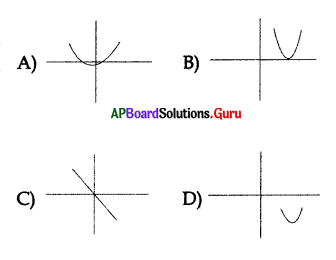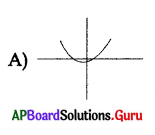Question 158.
If b2 = 4ac then the graph may be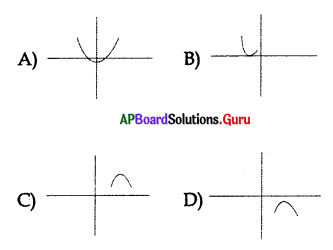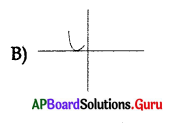Question 159.
The graph of a linear polynomial may be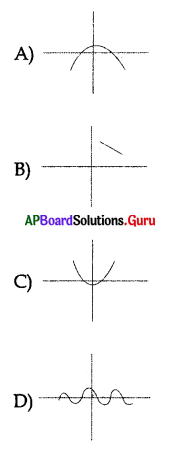Question 160.
Identify parabola among the following graphs.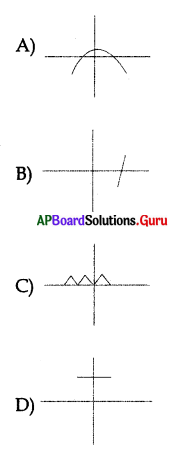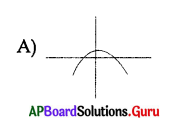Question 161.
If a quadratic equation have no real roots then the graph may be ………………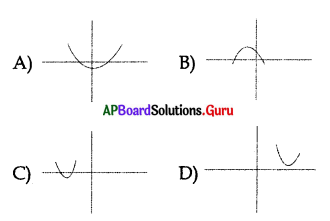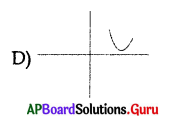Question 162.
If the graph of ax2 + bx + c = 0, a ≠ 0 never intersects X axis then the number of real zeroes are ………………..
A) 2
B) 0
C) 3
D) 4